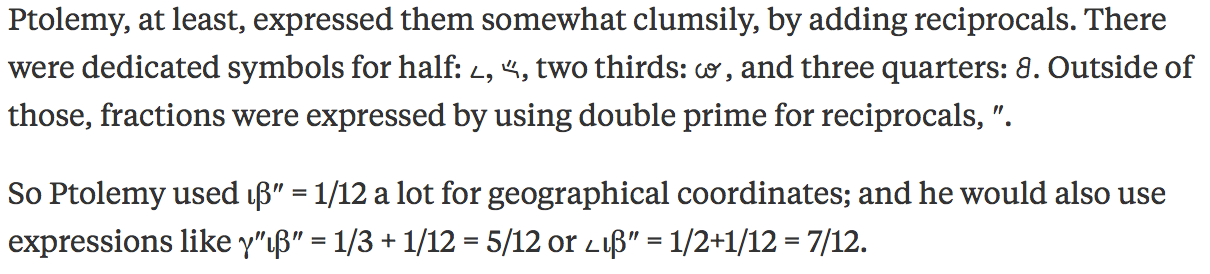# How did the Greeks represent fractions?

Ptolemy, at least, expressed them somewhat clumsily, by adding reciprocals. There were dedicated symbols for half: [math]unicode{x10175}, unicode{x10176}[/math], two thirds: [math]unicode{x10177}[/math], and three quarters: [math]unicode{x10178}.[/math] Outside of those, fractions were expressed by using double prime for reciprocals, ″.

So Ptolemy used ιβ″ = 1/12 a lot for geographical coordinates; and he would also use expressions like γ″ιβ″ = 1/3 + 1/12 = 5/12 or [math]unicode{x10175}[/math]ιβ″ = 1/2+1/12 = 7/12.

EDIT: For those without font support for Ancient Greek Numerals: